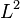# Regularization

## Definition

Regularization is described as follows.

We have:

• A set of features that predict an output value
• A set of training data, including the set of features and the output value
• A functional form, in terms of unknown parameters, that describes the output value in terms of the features. These parameters are also sometimes known as model weights.
• A choice of cost function (or error function) that measures the error for a given pair of predicted output and actual output.

Regularization refers to a process where we modify the cost function by adding to it an expression that captures the complexity of the feature set. The expression is typically a product of a hyperparameter (subject to smart hyperparameter optimization) and a fixed function of the parameters being learned (i.e., the model weights) chosen based on the problem domain. This fixed function is typically the$L^1$-norm, squared$L^2$-norm, or$L^\infty$-norm of the feature vector (sometimes with some coordinates removed).

Note that the choice of regularization, including the choice of hyperparameter, need to be known by the learning algorithm. Moreover, some learning algorithms that work for unregularized problems may not work for regularized problems, or may need to be modified to tackle the version with regularization.

Regularization is used only on the training data, not on test data that was withheld from the learning algorithm.

### Goal: enforcing simplicity and reducing complexity

Regularization introduces a penalty for complexity, and forces the parameter vector to be simple. This reduces the extent of overfitting.

Regularization can also enforce unique solutions in the case of overdetermined problems.

## Hyperparameter optimization for the regularization hyperparameter

Further information: hyperparameter optimization

Ideally, we would like to choose a regularization hyperparameter such that the parameters found by the model do best on new data that was withheld from the learning algorithm. The approach used for this is cross-validation: we cordon off a part of the training set from the learning algorithm (this cordoned-off part is called the cross-validation set), run the learning algorithm for different choices of hyperparameter, and compare the performance of all the solutions obtained on the cross-validation set. We pick the one that does best on this set and then check that it did well on the training set.

Some algorithms use the test set as their cross-validation set. For sufficiently large data sets, this is not a problem. However, for small and intermediate-sized data sets, this is problematic because we end up overfitting the regularization parameter itself by exposing it to influence from the test set. The neatest approach is to keep the cross-validation and test sets separate.